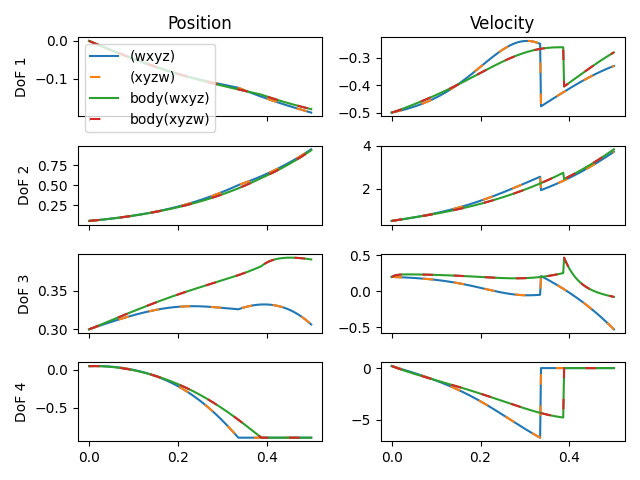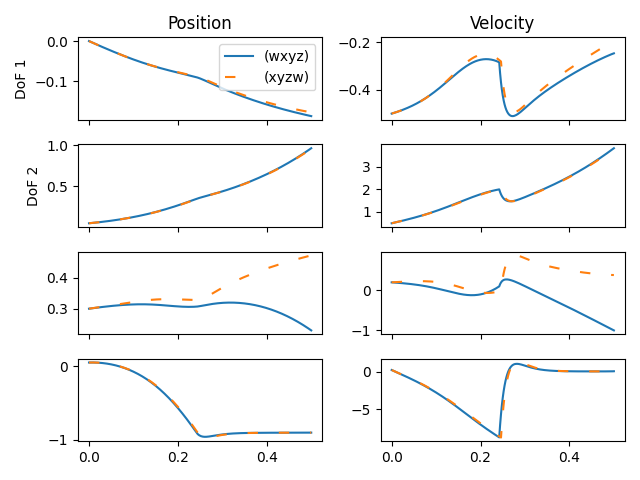# Isaac Sim Inertia Treatment

Dear Isaac Sim Team,

I recently ported a MuJoCo model to Isaac Sim using the available importer. I noticed that the off-diagonal elements were simply ignored, so I added the information about the off-diagonal elements in the USD file via the physics:principalAxes element of the PhysicsMassAPI.

Given that I was unsure whether I needed to present the quaternion in (w,x,y,z) or (x,y,z,w) convention, I simply tried both and looked at the simulation results. To my surprise, the results were the same.

Intrigued by this observation and thinking that Isaac Sim simply ignores off-diagonal inertia elements, I decided to side-step the physics:principalAxes element and create an xForm element that carries the diagonal inertia information and is rotated to align with the inertia frame. As an example, here is the conversion I did:

``````point3f physics:centerOfMass = (-0.0044, -0.0007, -0.12190001)
float3 physics:diagonalInertia = (0.09044195, 0.113096856, 0.1350886)
quat physics:principalAxes = (0.5153317, 0.48197561, 0.53200862, 0.46808074)
float physics:mass = 10.767688
``````
``````def Xform "inertia_properties" (
apiSchemas = ["PhysicsMassAPI"]
)
{
point3f physics:centerOfMass = (-0.0044, -0.0007, -0.12190001)
float3 physics:diagonalInertia = (0.09044195, 0.113096856, 0.1350886)
float physics:mass = 10.767688
quatd xformOp:orient = (0.5153317, 0.48197561, 0.53200862, 0.46808074)
double3 xformOp:translate = (0., 0., 0.)
uniform token[] xformOpOrder = ["xformOp:translate", "xformOp:orient"]
}
``````

This led to a difference compared to the previous simulations. However, the simulation was again indifferent to whether I specified the rotation in (w,x,y,z) or (x,y,z,w) convention.For the MuJoCo simulation, using the different quaternions made a noticeable difference in simulation outcome.So I wonder, is Isaac Sim treating the off-diagonal elements at all? And how are nested bodies treated by the simulator?

A short update: I realized that my issue may be very well related to this thread.

I already confirmed that indeed, Isaac Sim ignores the off-diagonal elements when importing the USD model by calling the “get_body_inertias()” method of the ArticulationView.

I will in the following days check whether I can, before starting the simulation, simply set the inertias with the “set_body_inertias()” method. However, I am afraid that this might not work since the Domain Randomization Page of IsaacSim only allows for the randomization of diagonal inertia elements and also only when simulating on the CPU (which I am not doing).

I have tried in many ways to make the inertia tensor correctly set in Isaac Sim but without any success.

The only way may be waiting for the team to fix this issue in the next release…

Hi @AlanSunHR,

funnily enough, I may have just fixed the problem (at least in my case).

While I can confirm that I was not able to programmatically set the inertia tensors (neither via set_body_coms nor set_body_inertias), I found an issue in my USDA scene description.

More precisely, I specified the principal axis of the inertia tensor as follows

``````quat physics:principalAxes = (0.5153317, 0.48197561, 0.53200862, 0.46808074)
``````

Turns out that the MassAPI specification wants

``````quatf physics:principalAxes = (0.5153317, 0.48197561, 0.53200862, 0.46808074)
``````

i.e. a quatf instead of a quat. Fixing this for all my bodies that use the MassAPI leads to the correct inertia tensors appearing after loading the model.

Maybe this also works in your case.

Best
Pascal

Hi there, thanks for raising the issue and sharing your solutions. The MJCF importer is not currently taking inertia elements into consideration. We will work on improving this feature for the next release.

I tried your method of add principleAxes in the USDA file. It correctly displayed the principle axes in Isaac Sim UI, but when I get the inertia matrix from the api ArticulationView.get_body_inertias (Core [omni.isaac.core] — isaac_sim 2022.2.1-beta.29 documentation), the calculated inertia matrices are still not correct.

I don’t know if the inertia matrices can be ignored or not, since we don’t know in the simulation backend, if only the principle axes and diagonal inertia are used.

But thanks any way for this workaround! At least we can make sure in the UI that the inertia is correct, and hope the team will fix the inertia issue soon.